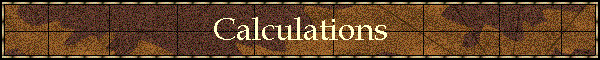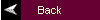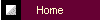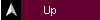## Calculations used in our performance data.

### Daily Gain

This is how we calculate the Daily Gain of an animal:

Daily Gain = (Weaning Weight - Birth Weight) / (Age at Weighing)
Where the Age at Weighing is very close to 205 days.

The birth weight of the calf is removed because in such a short time period the 85lb - 100lb that the calf is born with makes a big difference to the daily gain, also the calf did not gain the 85lb - 100lb after it was born.  Statistics can be twisted any way you want but this is the fairest way to do things. If the birth weight is not know we use a value of 85lb.

For example let us say that we have an 800lb calf with a 95lb birth weight = 705lb of weight gained since birth.  Let us say the calf was born Jan. 2, and weighed on Jul 31 thus it is 210 days old so:
(Weaning Weight - Birth Weight) / Age of Calf at Weaning  = Daily Gain
(800lb - 95lb) / 210days = 3.36lb gained per day

Here is an example of the difference of not removing the birth weight:
800lb / 210 days = 3.81lb/day
This would give 0.45lb/day more gain. The younger the animal the more profound the difference would be.

To find the 205 day adjusted weight you take the daily gain from  the weight closest to 205 days and multiply by 205 and add the birth weight.

Adjusted 205 Day Weight. = (Daily Gain * 205 days) + Birth Weight

For example: (3.36lb/day * 205days) + 95lb = 783.8lb Adjusted 205 Day Weight

### WPDA

To find the Weight Per Day Of Age (WPDA) of an animal, you take the weight at weighing very nearly or exactly at 365 days of age and divide by the actual age in days.  No deduction is made for birth weight in these numbers as the effect is negligible by this age:

WPDA = (Yearling Weight) / (Age at Weighing)
Where the Age at Weighing is very close to 365 days.

For example: 1200lb / 370 days = 3.24lb of weight per day gained.©1997-2000 Braunvieh International All rights reserved  Last Updated: 18-Nov-2001